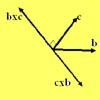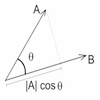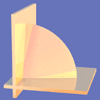# Resources tagged with: Vector products

There are 5 NRICH Mathematical resources connected to Vector products, you may find related items under Vectors and Matrices.

Broad Topics > Vectors and Matrices > Vector products### Multiplication of Vectors

##### Age 16 to 18

An account of multiplication of vectors, both scalar products and vector products.### Cross with the Scalar Product

##### Age 16 to 18Challenge Level

Explore the meaning of the scalar and vector cross products and see how the two are related.### Quaternions and Reflections

##### Age 16 to 18Challenge Level

See how 4 dimensional quaternions involve vectors in 3-space and how the quaternion function F(v) = nvn gives a simple algebraic method of working with reflections in planes in 3-space.### Walls

##### Age 16 to 18Challenge Level

Plane 1 contains points A, B and C and plane 2 contains points A and B. Find all the points on plane 2 such that the two planes are perpendicular.### V-P Cycles

##### Age 16 to 18Challenge Level

Form a sequence of vectors by multiplying each vector (using vector products) by a constant vector to get the next one in the seuence(like a GP). What happens?